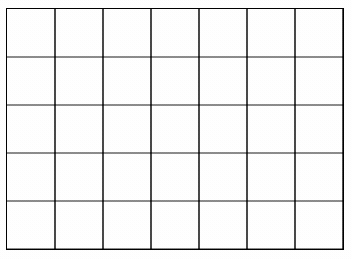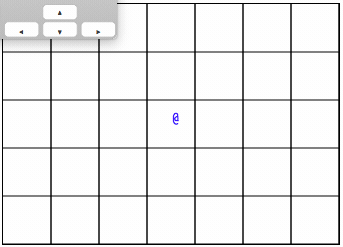## Super Mario Bros. 3 Overworld Map (Part 1)

This series is about writing a clone of the Super Mario Bros. 3 Overworld Map in javascript.Our end goal is to have a "playable" clone of the overworld map. We should be able to walk around using roads, to mark levels as completed, and be unable to access blocked areas.

Additionally, we want to have a way to generate maps dynamically given a definition file. That file would specify what we want in our map. For example, how many levels, which roads should be blocked by a door, what order the levels can be solved in, etc.

## Rendering on a canvas

Our first goal will be to display a grid and an icon representing the player.

For this, we will need a base class that manages a canvas element.

`WIDTH` is going to be the horizontal number of tiles, and `HEIGHT the vertical number of tiles.

``````class App {
constructor () {
this.el = document.createElement('canvas')
this.ctx = this.el.getContext('2d')

this.el.width = TILE_SIZE * WIDTH
this.el.height = TILE_SIZE * HEIGHT
this.el.style = 'border: 1px solid black;'

document.body.appendChild(this.el)
}
}``````

The next step is to add some rendering code. Let's add a method to our class for this.

``````App.prototype.render = function () {
this.ctx.fillStyle = 'white'
this.ctx.fillRect(0, 0, this.el.width, this.el.height)

for (let x = 0; x < WIDTH; x++) {
for (let y = 0; y < HEIGHT; y++) {
this.drawGrid(x, y)
}
}
}``````

We simply loop through all the tiles in our grid, and use the coordinates of each tile to call a method that draws that tiles grid lines. We also make sure to clear the canvas by filling it with a single color every time the `render` method is called.

``````App.prototype.drawGrid = function (x, y) {
this.ctx.fillStyle = 'black'
this.ctx.beginPath()
this.ctx.moveTo(x * TILE_SIZE, y * TILE_SIZE)
this.ctx.lineTo(x * TILE_SIZE + TILE_SIZE, y * TILE_SIZE)
this.ctx.lineTo(x * TILE_SIZE + TILE_SIZE, y * TILE_SIZE + TILE_SIZE)
this.ctx.stroke()
}``````

Drawing a tile's grid lines involves position the canvas' "pen" at the top-right corner and drawing first a first line towards the bottom-right corner, and then a second line from that point to the bottom-left corner.## Player Movement

Now, let's add a representation of the player:

``````constructor () {
// ...

this.hero = { x: 0, y: 0 }
}``````

This simple object will keep track of where the player is located.

Next we want to respond to player input:

``````App.prototype.setupListeners = function () {
if (e.key === 'ArrowUp') this.move(0, -1)
if (e.key === 'ArrowRight') this.move(1, 0)
if (e.key === 'ArrowDown') this.move(0, 1)
if (e.key === 'ArrowLeft') this.move(-1, 0)
})
}``````

The keydown event tells us that the player pressed down on a key. We use the payload from that event (at the `key` field) to know what keyboard key that was. Based on that information, we can pass a directional vector to our `move` method. A value of `0` means that no change will happen in that direction, `-1` means up in the y-axis and left on the x-axis, while a value of `1` means down on the y-axis and right on the x-axis.

``````App.prototype.move = function (x, y) {
this.hero.x = Math.min(WIDTH - 1, Math.max(0, this.hero.x + x))
this.hero.y = Math.min(HEIGHT - 1, Math.max(0, this.hero.y + y))

this.render()
}``````

Our `move` method takes the directional vector and modifies the player's coordinates, making sure that the final destination is not out of bounds with the map's outer edges. It's a simple case of adding the value that was passed to the method with the appropriate variable and comparing the result with either 0 (which represents the upper and left edges) or the value of the constants we used for the number of tiles in our grid (which represent the lower and right edges).

The only part missing is to render an icon that represents the player. For now, let's just use the '@' symbol.

``````App.prototype.drawHero = function () {
this.ctx.fillStyle = 'blue'
this.ctx.fillText(
'@',
this.hero.x * TILE_SIZE + HALF_TILE,
this.hero.y * TILE_SIZE + HALF_TILE
)
}``````

Remember to call this function in the render method!And now we have player movement.

In the next part, we'll look at adding a transition when the player is moving between tiles, and begin to look at some logic around tile accessibility.

I don't have a newsletter right now.